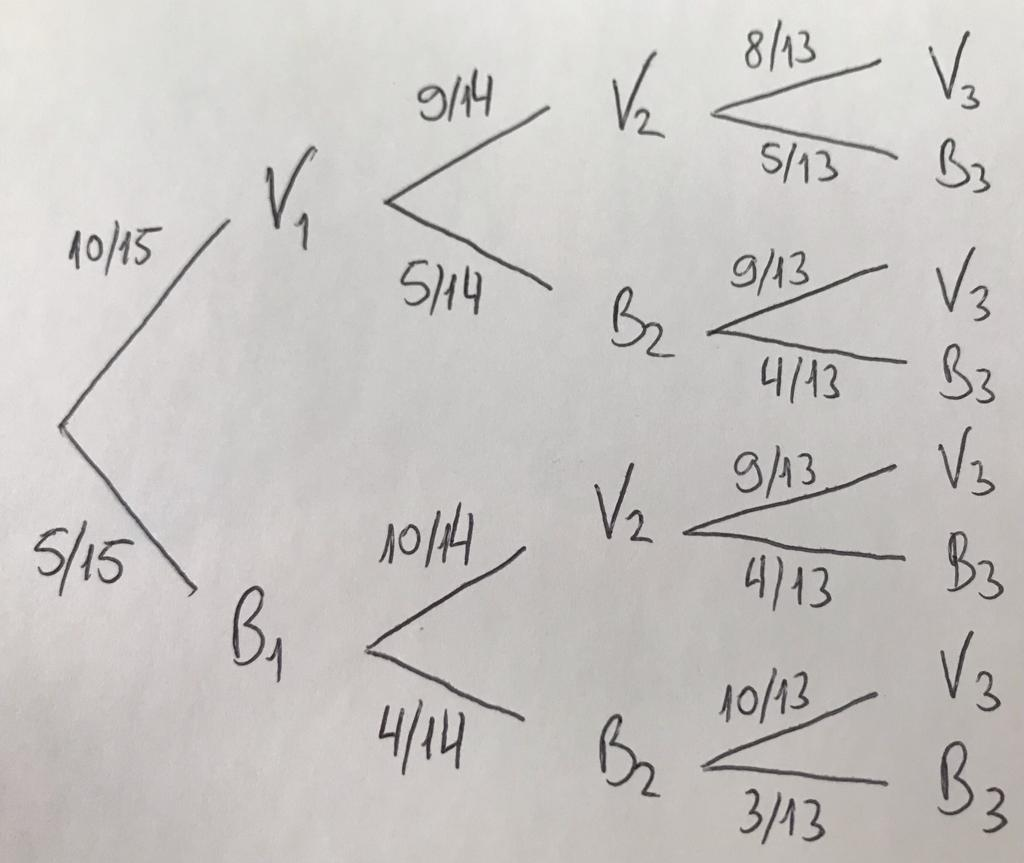## 5.2 Exchangeability

Exchangeability is essentially an expression of symmetry in the probabilistic behaviour of events.

Definition 5.1 Exchangeability by . The operations $$R$$ and $$S$$ are interchangeable: $$RS=SR$$, and therefore $$R^r S^s = S^s R^r = S^{s_1} R^{r_1} S^{s_2} R^{r_2} \ldots \;\;$$ ($$s_1+s_2+\ldots = s$$, $$r_1+r_2+\ldots = r$$).

Definition 5.2 Exchangeability by . Given $$n$$ events, the probabilities (…) that $$h$$ of them will occur ($$h = 0,1,\ldots,n$$) are now arbitrary. (…) However, the combinations of $$h$$ 1s and $$n-h$$ 0s all have the same probability.

Example 5.2 Consider a random sample without replacement of marbles from an urn. The composition is known, with 10 red (V) and 5 white (B) balls. The balls are selected one by one and the event $$V_i$$ is defined to occur when the $$i$$-th ball sampled is red. Similarly, $$B$$ can be used for the event related to the white ball.The probabilities can be calculated from the tree diagram above.

\begin{align*} Pr(V_1) &= \frac{10}{15} = \frac{2}{3} \\ Pr(V_2) &= \frac{10}{15}\cdot\frac{9}{14} + \frac{5}{15}\cdot\frac{10}{14} = \frac{2}{3} \\ Pr(V_3) &= \frac{10}{15}\cdot\frac{9}{14}\cdot\frac{8}{13} + \frac{10}{15}\cdot\frac{5}{14}\cdot\frac{9}{13} + \frac{5}{15}\cdot\frac{10}{14}\cdot\frac{9}{13} + \frac{5}{15}\cdot\frac{4}{14}\cdot\frac{10}{13} = \frac{2}{3} \\ Pr(V_1 V_2) &= \frac{10}{15}\cdot\frac{9}{14} = \frac{3}{7} \\ Pr(V_2 V_3) &= \frac{10}{15}\cdot\frac{9}{14}\cdot\frac{8}{13} + \frac{5}{15}\cdot\frac{10}{14}\cdot\frac{9}{13} = \frac{3}{7} \\ Pr(V_1 V_3) &= \frac{10}{15}\cdot\frac{9}{14}\cdot\frac{8}{13} + \frac{10}{15}\cdot\frac{5}{14}\cdot\frac{9}{13} = \frac{3}{7} \\ Pr(V_2|V_1) &= \frac{9}{14} \end{align*}

As $$Pr(V_2|V_1) = \frac{9}{14} \ne \frac{2}{3} = Pr(V_2)$$, $$V_1$$ and $$V_2$$ are not independent, i.e., knowledge about the occurrence of $$V_1$$ changes the probability of $$V_2$$.

Note that the probabilities $$P(V_i)$$ are equal to 10/15, for $$i \in \{1,2,3\}$$. Furthermore, the intersection probabilities are such that $$Pr(V_i V_j) = 3/7$$ for $$i,j \in \{1,2,3\}$$, $$i \ne j$$. This happens because the aforementioned events are exchangeable. This is a well-known example of dependency, with exchangeability: the selections are indistinguishable, but still dependent.

Example 5.3 Again with the data from Example 5.2, withdrawals can be considered with replacement. Thus,

\begin{align*} Pr(V_1) &= Pr(V_2) = Pr(V_3) = \frac{10}{15} = \frac{2}{3} \\ Pr(V_1 V_2) &= Pr(V_2 V_3) = Pr(V_1 V_3) = \frac{4}{9} \\ Pr(V_2|V_1) &= \frac{10}{15} = \frac{2}{3} \end{align*}

In this example, we obtain the same probabilities $$Pr(V_1)$$, $$Pr(V_2)$$ and $$Pr(V_3)$$ as in Example 5.2. The conditional probabilities, however, are now equal to the unconditional probabilities, for example, $$Pr(V_2|V_1) = P(V_2)$$. This is therefore a situation in which events are not only exchangeable, but also independent.

It is worth mentioning that in both examples above the urn has a known composition. If the composition is unknown, the events are only exchangeable but not independent, even when draws are made with replacement.

### References

Barnett, Vic. 1999. Comparative Statistical Inference. John Wiley & Sons. https://onlinelibrary.wiley.com/doi/book/10.1002/9780470316955.
Cordani, Lisbeth K, and Sergio Wechsler. 2006. “Teaching Independence and Exchangeability.” In Proceedings of the 7th International Conference on Teaching Statistics. Salvador, Brasil: International Association for Statistics Education. https://www.stat.auckland.ac.nz/~iase/publications/17/3I1_CORD.pdf.
Finetti, Bruno de. 1930. “rFunzione Caratteristica Di Un Fenomeno Aleatorio.” Accademia Nazionale Dei Lincei, Classe Di Scienze Fisiche, Matematiche e Naturali IV: 86–133. http://www.brunodefinetti.it/Opere/funzioneCaratteristica.pdf.
———. 1974. “Theory of Probability. A Critical Introductory Treatment, 2 Volumes. Translation by a. Machi and a.f.m. Smith of 1970 Book.” Wiley ISBN.